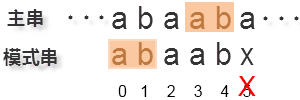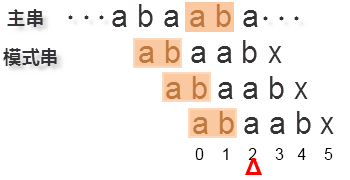0
3
01. 云栖社区>
2. 博客>
3. 正文

每周一算法之六——KMP字符串匹配算法

nothingfinal 2018-03-09 12:01:00 浏览460

KMP是一种著名的字符串模式匹配算法，它的名称来自三个发明人的名字。这个算法的一个特点就是，在匹配时，主串的指针不用回溯，整个匹配过程中，只需要对主串扫描一遍就可以了。因此适合对大字符串进行匹配。

算法的原理next[j] = Max{ k | 0<=k<j 并且 'patten0...pattenk-1' = 'pattenj-k...pattenj-1' }

主串匹配算法

static int Kmp(String s, String patten) {
int i = 0, j = -1;
int[] next = GetNext(patten);// 待实现

while (i < s.length() && j < patten.length()) {
if (j == -1 || s.charAt(i) == patten.charAt(j)) {
i++;
j++;
} else {
j = next[j];// 失配时跳转
}
}

if (j == patten.length()) // 完全匹配
return i - j;

return -1;
}

next是恒为-1的，所以如果在下标0处失配，则下一次循环j等于-1，i就会在循环中指向下一个字符，j也恢复为0。

模式串的next数组生成算法static int[] GetNext(String s) {
int i = 0, j = -1;
int[] next = new int[s.length()];
next = -1; // 这个初始化时必须的

while( i<s.length()-1)
{
if( j == -1 || s.charAt(i) == s.charAt(j))
{
i++;
j++;
next[i] = j;
}
else
{
j = next[j];// 当j在下标零处失配，代码会怎么执行呢？
}
}
return next;
}

nothingfinal
+ 关注